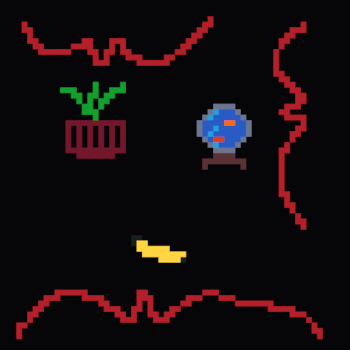## nightly discrete_range_map

This crate provides DiscreteRangeMap and DiscreteRangeSet, Data Structures for storing non-overlapping discrete intervals based off BTreeMap

### 6 releases

 0.5.1 Jul 1, 2023 Jul 1, 2023 Jun 17, 2023 May 16, 2023 Apr 24, 2023

#162 in Data structures

Used in gap_query_interval_tree

AGPL-3.0-or-later

96KB
2K SLoC

# discrete_range_mapThis crate provides `DiscreteRangeMap` and `DiscreteRangeSet`, Data Structures for storing non-overlapping discrete intervals based off `BTreeMap`.

## You must implement `Copy`

Due to implementation complications with non-`Copy` types the datastructures currently require both the range type and the points the ranges are over to be `Copy`.

## Example using an Inclusive-Exclusive range

``````use discrete_range_map::test_ranges::ie;
use discrete_range_map::DiscreteRangeMap;

let mut map = DiscreteRangeMap::new();

map.insert_strict(ie(0, 5), true);
map.insert_strict(ie(5, 10), false);

assert_eq!(map.overlaps(ie(-2, 12)), true);
assert_eq!(map.contains_point(20), false);
assert_eq!(map.contains_point(5), true);
``````

## Example using a custom range type

``````use discrete_range_map::test_ranges::ie;
use discrete_range_map::{
DiscreteFinite, Interval, DiscreteRangeMap,
FiniteRange,
};

#[derive(Debug, Copy, Clone)]
enum Reservation {
// Start, End (Inclusive-Exclusive)
Finite(i8, i8),
// Start (Inclusive-Forever)
Infinite(i8),
}

// First, we need to implement FiniteRange
impl FiniteRange<i8> for Reservation {
fn start(&self) -> i8 {
match self {
Reservation::Finite(start, _) => *start,
Reservation::Infinite(start) => *start,
}
}
fn end(&self) -> i8 {
match self {
//the end is exclusive so we take off 1 with checking
//for compile time error overflow detection
Reservation::Finite(_, end) => end.down().unwrap(),
Reservation::Infinite(_) => i8::MAX,
}
}
}

// Second, we need to implement From<Interval<i8>>
impl From<Interval<i8>> for Reservation {
fn from(bounds: Interval<i8>) -> Self {
if bounds.end == i8::MAX {
Reservation::Infinite(bounds.start)
} else {
Reservation::Finite(
bounds.start,
bounds.end.up().unwrap(),
)
}
}
}

// Next we can create a custom typed DiscreteRangeMap
let reservation_map = DiscreteRangeMap::from_slice_strict([
(Reservation::Finite(10, 20), "Ferris".to_string()),
(Reservation::Infinite(20), "Corro".to_string()),
])
.unwrap();

for (reservation, name) in reservation_map.overlapping(ie(16, 17))
{
println!(
"{name} has reserved {reservation:?} inside the range 16..17"
);
}

for (reservation, name) in reservation_map.iter() {
println!("{name} has reserved {reservation:?}");
}

assert_eq!(
reservation_map.overlaps(Reservation::Infinite(0)),
true
);
``````

## Key Understandings and Philosophies:

### Discrete-ness

This crate is designed to work with `Discrete` types as compared to `Continuous` types. For example, `u8` is a `Discrete` type, but `String` is a `Continuous` if you try to parse it as a decimal value.

The reason for this is that common `interval-Mathematics` operations differ depending on wether the underlying type is `Discrete` or `Continuous`. For example `5..=6` touches `7..=8` since integers are `Discrete` but `5.0..=6.0` does not touch `7.0..=8.0` since the value `6.5` exists.

### Finite-ness

This crate is also designed to work with `Finite` types since it is much easier to implement and it is not restrictive to users since you can still represent `Infinite` numbers in `Finite` types paradoxically using the concept of `Actual Infinity`.

For example you could define `Infinite` for `u8` as `u8::MAX` or if you still want to use `u8::MAX` as a `Finite` number you could define a wrapper type for `u8` that adds an `Actual Infinity` value to the `u8` set.

### Invalid Ranges

Within this crate, not all ranges are considered valid ranges. The definition of the validity of a range used within this crate is that a range is only valid if it contains at least one value of the underlying domain.

For example, `4..6` is considered valid as it contains the values `4` and `5`, however, `4..4` is considered invalid as it contains no values. Another example of invalid range are those whose start values are greater than their end values. such as `5..2` or `100..=40`.

Here are a few examples of ranges and whether they are valid:

range valid
0..=0 YES
0..0 NO
0..1 YES
9..8 NO
(Bound::Exluded(3), Bound::Exluded(4)) NO
400..=400 YES

### Overlap

Two ranges are "overlapping" if there exists a point that is contained within both ranges.

### Touching

Two ranges are "touching" if they do not overlap and there exists no value between them. For example, `2..4` and `4..6` are touching but `2..4` and `6..8` are not, neither are `2..6` and `4..8`.

### Merging

When a range "merges" other ranges it absorbs them to become larger.

See Wikipedia's article on mathematical Intervals: https://en.wikipedia.org/wiki/Interval_(mathematics)

# Credit

I originally came up with the `StartBound`: `Ord` bodge on my own, however, I later stumbled across `rangemap` which also used a `StartBound`: `Ord` bodge. `rangemap` then became my main source of inspiration.

Later I then undid the `Ord` bodge and switched to my own full-code port of `BTreeMap`, inspired and forked from `copse`, for it's increased flexibility.

# Origin

The aim for this library was to become a more generic superset of `rangemap`, following from this issue and this pull request in which I changed `rangemap`'s `RangeMap` to use `RangeBounds`s as keys before I realized it might be easier and simpler to just write it all from scratch.

It is however worth noting the library eventually expanded and evolved from it's origins.

This crate was previously named `range_bounds_map`.

# Similar Crates

Here are some relevant crates I found whilst searching around the topic area:

~1.3–2MB
~41K SLoC